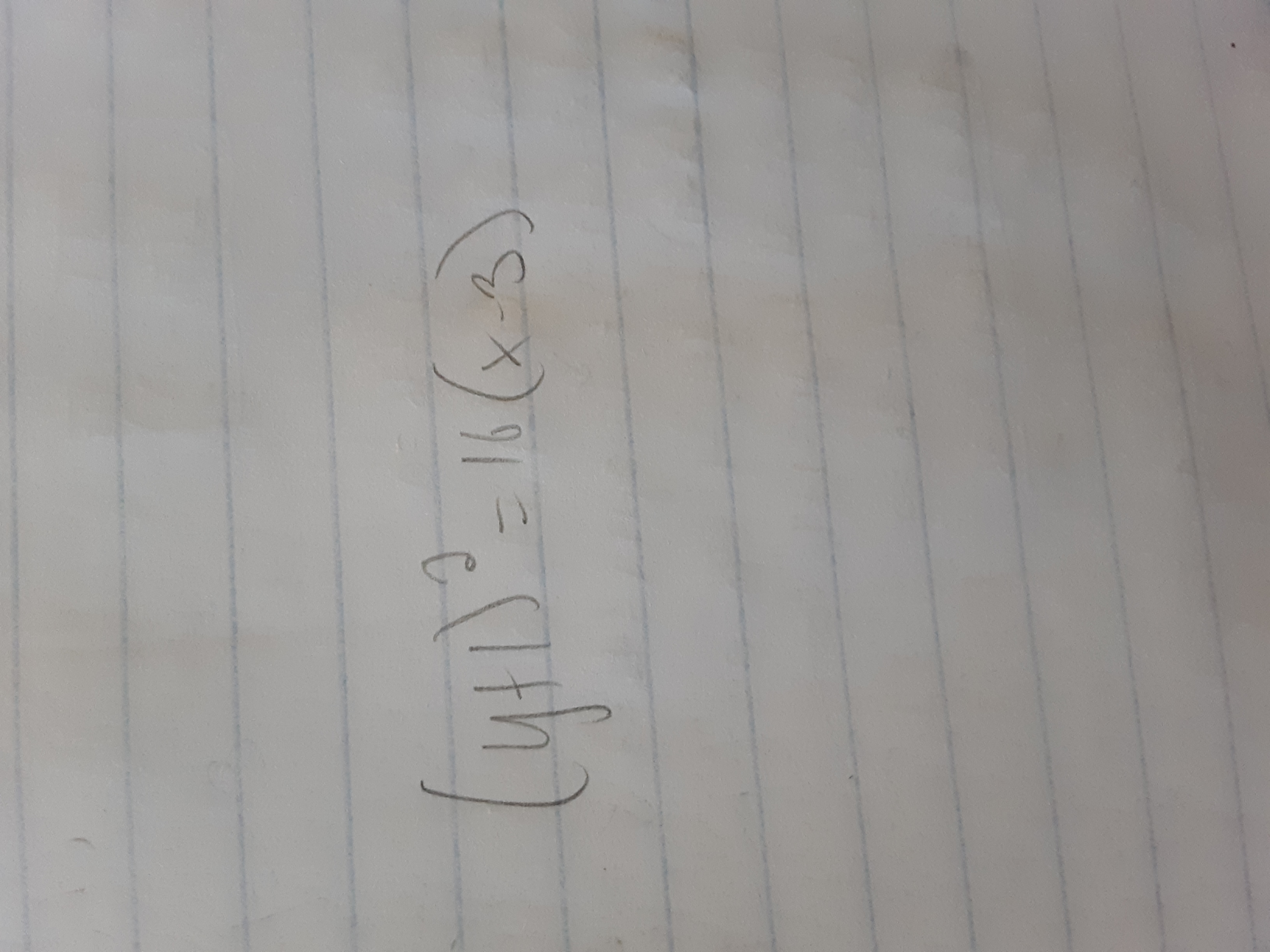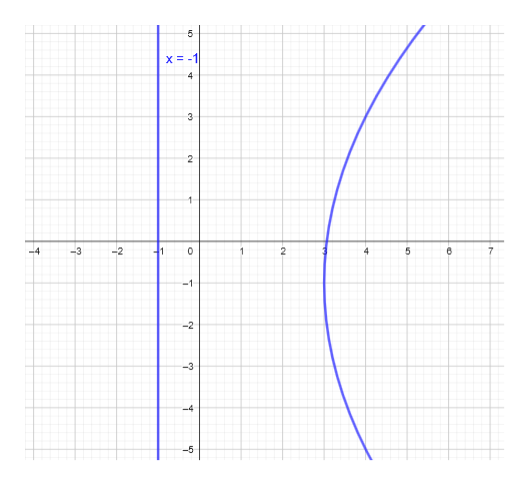# 2y46 013-20 Graphing Shifted Parabolas An equation of a parabolais given. (a) Find the vertex, focus, and directrix of the parabola.(b) Sketch a graph showing the parabola and its directrix

Question
1 viewshelp_outlineImage Transcriptionclose2y 46 0 13-20 Graphing Shifted Parabolas An equation of a parabola is given. (a) Find the vertex, focus, and directrix of the parabola. (b) Sketch a graph showing the parabola and its directrix fullscreenfullscreen
check_circle

star
star
star
star
star
1 Rating
Step 1
1. a) Given equation of parabola is

The standard equation of a right-left parabola with vertex at (h,k) and focal length p is given by:

Rewriting the given equation:

Here h = 3, k = -1 and p = 4

Hence the vertex is (h,k) = (3, -1)

And the focus is given by (h + p, k) = (3 + 4, -1) = (7,-1)

The directrix is given by x = hp

Hence, the directrix is x = 3 – 4 = -1

Step 2

b)

Plotting the graph using the ...help_outlineImage Transcriptionclose5 X-1 4 3 2 1 -4 -2 -1 0 2 5 -1 -2 -3 -4 -5 fullscreen

### Want to see the full answer?

See Solution

#### Want to see this answer and more?

Solutions are written by subject experts who are available 24/7. Questions are typically answered within 1 hour.*

See Solution
*Response times may vary by subject and question.
Tagged in

### Calculus